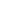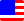Documents  >  Tutorial  >  group3
Operators of the third group

Purpose of this chapter.

We will learn about the multiplication operator, division operator, and remainder operator.

Operators of the third group.

Operators of the third group are following.

 * x * y multiplication operator / x / y division operator % x % y remainder operator

These are arithmetic operators with high priority.

multiplication operator

Multiplication operator is an operator for performing multiplication on the left and right operands of operator. The operand must be an instance of the int, long, or real class.

The class of the result depends on the class of the operand.

If there is an instance of the real class in the operand, the class of the result is real.

For example,

real r0 = 123.5 * 10.0; // The left is real and the right is real. Therefore, result is real.

real r1 = 123.5 * 10; // The left is real and the right is int. Therefore, result is real.

real r2 = 123.5 * 10L; // The left is real and right is long. Therefore, result is real.

If there is no real class in the operand and there is an instance of the long class, the class of the result is long.

For example,

long l0 = 123L * 10L; // The left is long and the right is long. Therefore, result is long.

long l1 = 123L * 10; // The left is long and the right is int. Therefore, result is long.

Otherwise, the class of the result is int.

For example,

real i0 = 123 * 10; // The left is int and the right is int. Therefore, result is int.

If the value of the result exceeds the range of the class, an exception is thrown.

For example,

int max = 0.Max;

int result = max * 2; // Over flow. Exception will be thrown.

The real literal does not overflow. However, the real as a result of the multiplication throws an exception due to overflow.

That is, if the result of the operation evaluates to infinity, an exception is thrown due to overflow.

division operator

Division operator is an operator that divides the left operand by the right operand. The operand must be an instance of the int, long, or real class.

The class of the result depends on the class of the operand.

If there is an instance of the real class in the operand, the class of the result is real.

For example,

real r0 = 123.5 / 10.0; // The left is real and the right is real. Therefore, result is real.

real r1 = 123.5 / 10; // The left is real and the right is int. Therefore, result is real.

real r2 = 123.5 / 10L; // The left is real and right is long. Therefore, result is real.

If there is no real class in the operand and there is an instance of the long class, the class of the result is long.

For example,

long l0 = 123L / 10L; // The left is long and the right is long. Therefore, result is long.

long l1 = 123L / 10; // The left is long and the right is int. Therefore, result is long.

Otherwise, the class of the result is int.

For example,

real i0 = 123 / 10; // The left is int and the right is int. Therefore, result is int.

If the result type is the int or long class, the remainder is truncated.

If division by zero, an exception is thrown.

If the value of the result exceeds the range of the class, an exception is thrown.

remainder operator

The remainder operator is an operator that divides the left operand by the right operand and find its remainder. The operand must be an instance of the int, long, or real class.

The class of the result depends on the class of the operand.

If there is an instance of the real class in the operand, the class of the result is real.

For example,

real r0 = 123.5 % 10.0; // The left is real and the right is real. Therefore, result is real.

real r1 = 123.5 % 10; // The left is real and the right is int. Therefore, result is real.

real r2 = 123.5 % 10L; // The left is real and right is long. Therefore, result is real.

If there is no real class in the operand and there is an instance of the long class, the class of the result is long.

For example,

long l0 = 123L % 10L; // The left is long and the right is long. Therefore, result is long.

long l1 = 123L % 10; // The left is long and the right is int. Therefore, result is long.

Otherwise, the class of the result is int.

For example,

real i0 = 123 % 10; // The left is int and the right is int. Therefore, result is int.

If division by zero, an exception is thrown.

If the value of the result exceeds the range of the class, an exception is thrown.